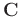## Including the structure-oriented space-varying median filter into an inversion framework

The blending process can be summarized as the following equation:(7)

whereis the blended data,is the blending operator, andis the unblended data. The formulation ofhas been introduced in Mahdad (2012) in detail. When considered in time domain,corresponds to blending different receiver signals into one channel according to the shot schedules of different shots. Deblending amounts to inverting equation 7 and recoveringfrom.

Because of the ill-posed property of this problem, all inversion methods require some constraints. Chen et al. (2014) proposed a general iterative deblending framework via shaping regularization Fomel (2007a). The iterative deblending is expressed as:(8)

whereis the shaping operator, which provides some constraints on the model, andis the backward operator, which approximates the inverse of. The shaping regularization framework offers us much freedom in constraining an under-determined problem by allowing different types of constraints. In this paper, the backward operator is simply chosen as, whereis a scale coefficient closely related with the blending fold, andstands for the adjoint operator of(or the pseudo-deblending operator). For example,can be optimally chosen asin a two-source dithering configuration (Chen et al., 2014; Mahdad, 2012).

Given a sparsity or rank constraint based shaping operator, we are proposing the hybrid shaping operator, which is constructed by cascading the structure-oriented space-varying median filter and the rank constraint based shaping operators. During the iterations, we need to vary the filter lengthwith each iteration, e.g., starting from a relatively largeand decreasing it to 1. It is worth noting that the median filter based methods can only be effective in filtering but not effective in inversion if not combined with other constraints. It is because the median filter is very powerful in rejecting impulsive noise but is not convenient to control for signal preservation.

2020-02-10